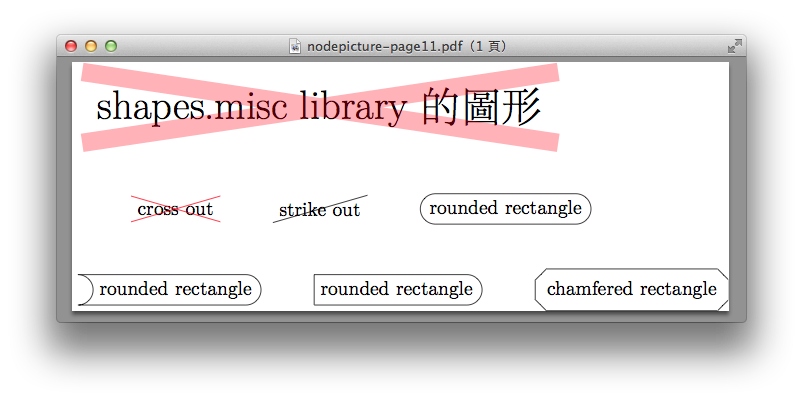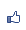Contents ...
udn網路城邦
TiKz 的 node 之略觀
2015/04/04 10:47

\node (a) at (0,3){};

\node (b) at(3,3){};

\node (c) at (3,2){};

\draw [red, thick](a)--(b)--(c)--(a);

\coordinate (A) at (0,1) coordinate (B) at (3,1) coordinate (C) at (3,0);

\draw [blue, thick](A)--(B)--(C)--(A);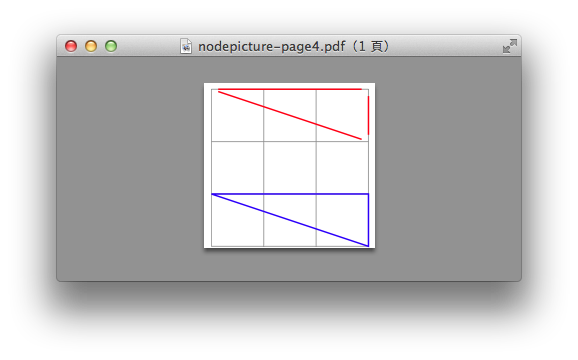\node [draw] (a) at (0,0){};

\node[draw, inner sep=0.3cm] (a) at (0,0){};

\node [draw,circle,inner step=5pt] (A) at (0,0){\$A\$};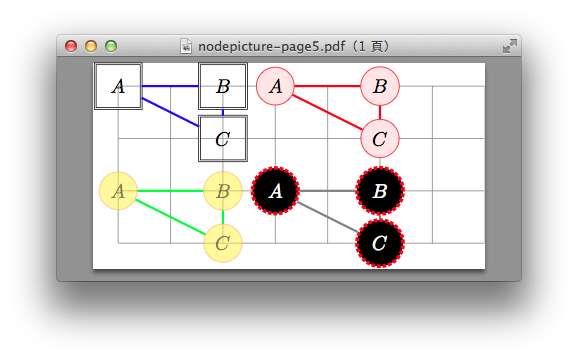\usetikzlibrary{shapes.geometric} :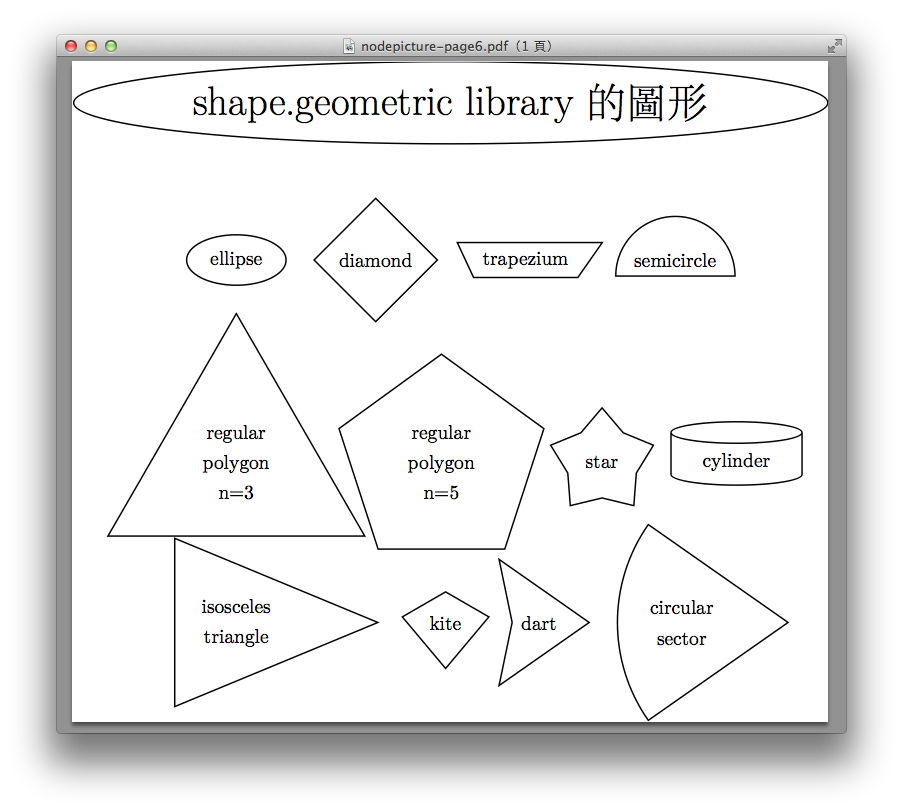\usetikzlibrary{shapes.symbols}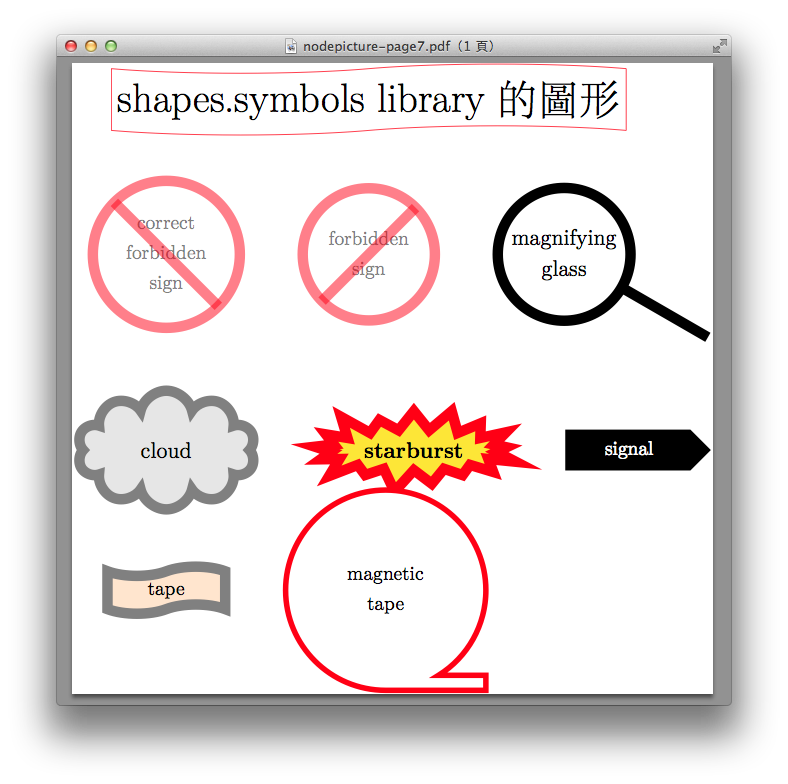\usetikzlibrary{shapes.arrows}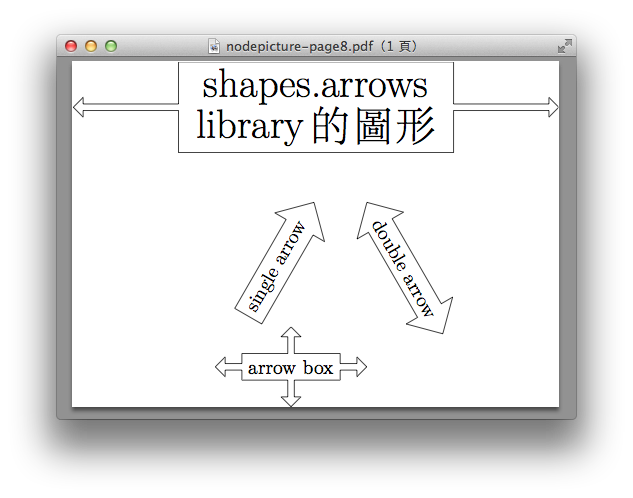\usetikzlibrary{shapes.multipart}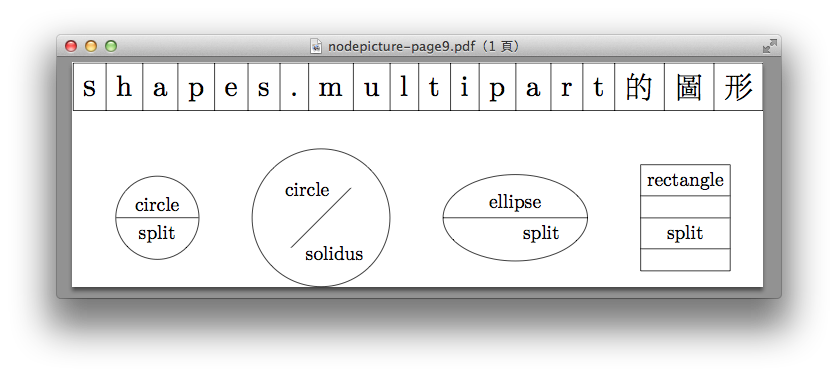\usetikzlibrary{shapes.callouts}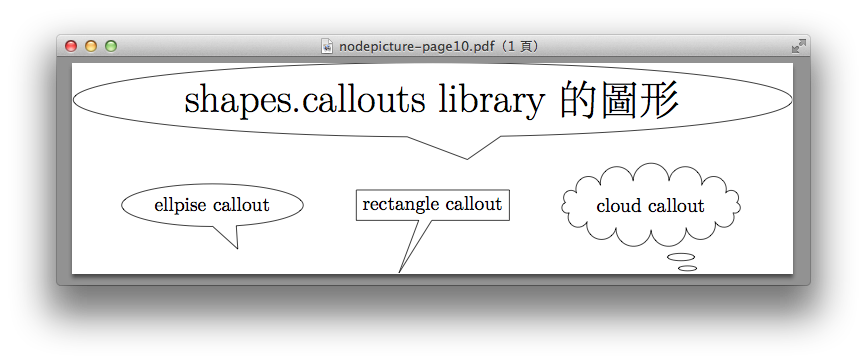\usetikzlibrary{shapes.misc}